# vector algebra - exercise on basics

12 de May de 2021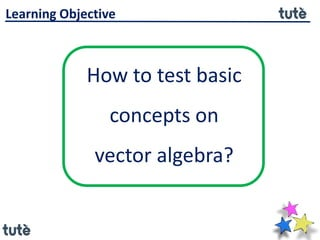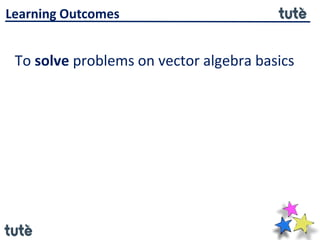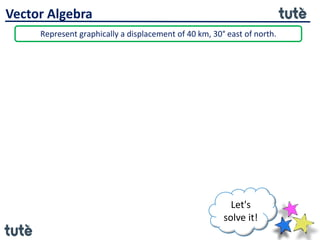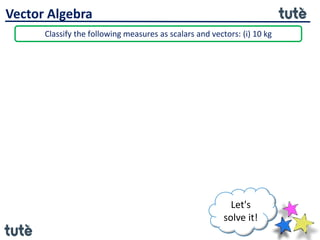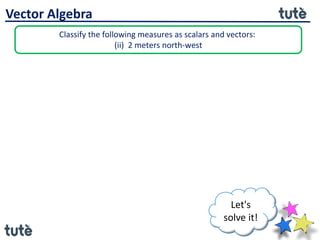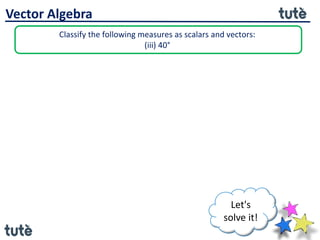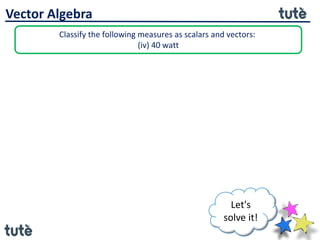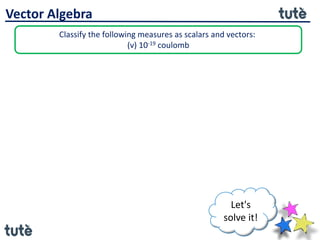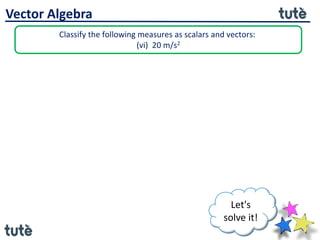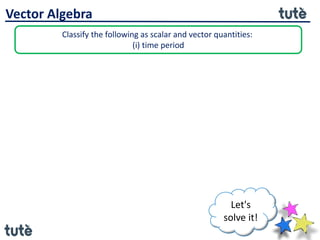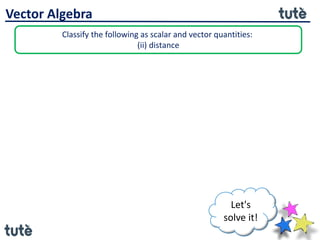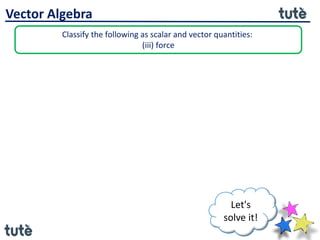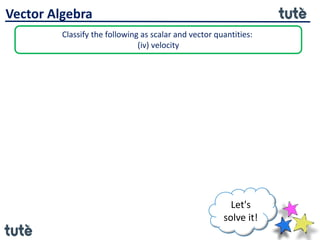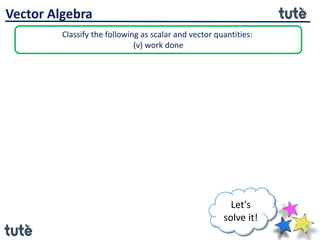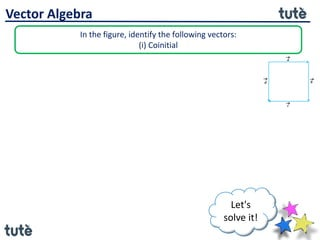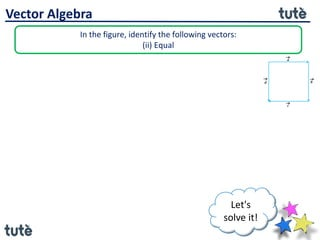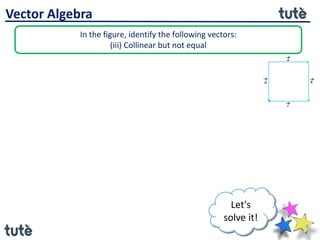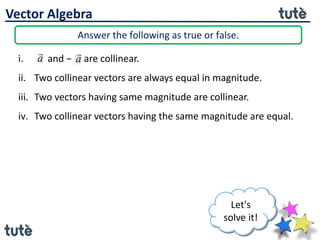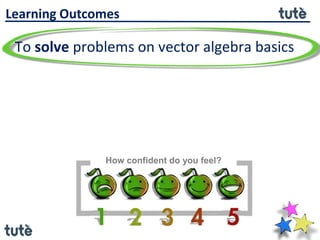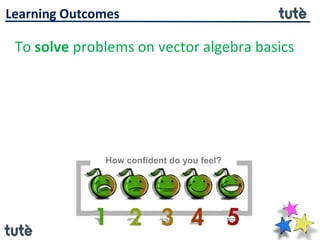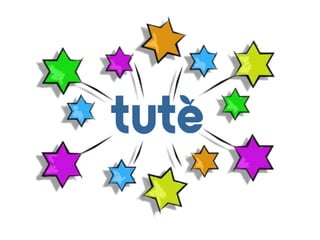1 de 22

### vector algebra - exercise on basics

1. How to test basic concepts on vector algebra? Learning Objective
2. To solve problems on vector algebra basics Learning Outcomes
3. Vector Algebra Represent graphically a displacement of 40 km, 30° east of north. Let's solve it!
4. Vector Algebra Classify the following measures as scalars and vectors: (i) 10 kg Let's solve it!
5. Vector Algebra Classify the following measures as scalars and vectors: (ii) 2 meters north-west Let's solve it!
6. Vector Algebra Classify the following measures as scalars and vectors: (iii) 40° Let's solve it!
7. Vector Algebra Classify the following measures as scalars and vectors: (iv) 40 watt Let's solve it!
8. Vector Algebra Classify the following measures as scalars and vectors: (v) 10-19 coulomb Let's solve it!
9. Vector Algebra Classify the following measures as scalars and vectors: (vi) 20 m/s2 Let's solve it!
10. Vector Algebra Classify the following as scalar and vector quantities: (i) time period Let's solve it!
11. Vector Algebra Classify the following as scalar and vector quantities: (ii) distance Let's solve it!
12. Vector Algebra Classify the following as scalar and vector quantities: (iii) force Let's solve it!
13. Vector Algebra Classify the following as scalar and vector quantities: (iv) velocity Let's solve it!
14. Vector Algebra Classify the following as scalar and vector quantities: (v) work done Let's solve it!
15. Vector Algebra In the figure, identify the following vectors: (i) Coinitial Let's solve it!
16. Vector Algebra In the figure, identify the following vectors: (ii) Equal Let's solve it!
17. Vector Algebra In the figure, identify the following vectors: (iii) Collinear but not equal Let's solve it!
18. Vector Algebra Answer the following as true or false. Let's solve it! i. A and − a are collinear. ii. Two collinear vectors are always equal in magnitude. iii. Two vectors having same magnitude are collinear. iv. Two collinear vectors having the same magnitude are equal.
19. To solve problems on vector algebra basics Learning Outcomes How confident do you feel?
20. To solve problems on vector algebra basics Learning Outcomes How confident do you feel?
21. How to test basic concepts on vector algebra? Learning Objective

### Notas del editor

1. An overview of the content of the lesson Must be in the form of a question where appropriate Students should be able to answer the question at the end - either fully, partly or in a way that demonstrates they understand what gaps in their knowledge they need to address Verbs such as to understand / to know / to gain confidence / to learn Ask students to give the question a go and point out that, at the end of the lesson, they should be able to answer fully
2. Measurable outcomes that students can demonstrate and self-assess against Must be written using Bloom’s taxonomy verbs Verbs based on students ability and pitch of lesson It must be clear that students understand the outcomes before moving on Make an activity of this slide: Ask students to read this aloud Ask them to paraphrase Ask that they explain what they mean Ask what they already know related to these outcomes There may be as few as 2 outcomes, or max 4
3. The next slides should be focused on achieving first outcome Make reference to the outcome in the teaching Fill this with thinking skills activities, peer assessment, higher-order questioning, engaging activities and challenge
4. The next slides should be focused on achieving first outcome Make reference to the outcome in the teaching Fill this with thinking skills activities, peer assessment, higher-order questioning, engaging activities and challenge
5. The next slides should be focused on achieving first outcome Make reference to the outcome in the teaching Fill this with thinking skills activities, peer assessment, higher-order questioning, engaging activities and challenge
6. The next slides should be focused on achieving first outcome Make reference to the outcome in the teaching Fill this with thinking skills activities, peer assessment, higher-order questioning, engaging activities and challenge
7. The next slides should be focused on achieving first outcome Make reference to the outcome in the teaching Fill this with thinking skills activities, peer assessment, higher-order questioning, engaging activities and challenge
8. The next slides should be focused on achieving first outcome Make reference to the outcome in the teaching Fill this with thinking skills activities, peer assessment, higher-order questioning, engaging activities and challenge
9. The next slides should be focused on achieving first outcome Make reference to the outcome in the teaching Fill this with thinking skills activities, peer assessment, higher-order questioning, engaging activities and challenge
10. The next slides should be focused on achieving first outcome Make reference to the outcome in the teaching Fill this with thinking skills activities, peer assessment, higher-order questioning, engaging activities and challenge
11. The next slides should be focused on achieving first outcome Make reference to the outcome in the teaching Fill this with thinking skills activities, peer assessment, higher-order questioning, engaging activities and challenge
12. The next slides should be focused on achieving first outcome Make reference to the outcome in the teaching Fill this with thinking skills activities, peer assessment, higher-order questioning, engaging activities and challenge
13. The next slides should be focused on achieving first outcome Make reference to the outcome in the teaching Fill this with thinking skills activities, peer assessment, higher-order questioning, engaging activities and challenge
14. The next slides should be focused on achieving first outcome Make reference to the outcome in the teaching Fill this with thinking skills activities, peer assessment, higher-order questioning, engaging activities and challenge
15. The next slides should be focused on achieving first outcome Make reference to the outcome in the teaching Fill this with thinking skills activities, peer assessment, higher-order questioning, engaging activities and challenge
16. The next slides should be focused on achieving first outcome Make reference to the outcome in the teaching Fill this with thinking skills activities, peer assessment, higher-order questioning, engaging activities and challenge
17. The next slides should be focused on achieving first outcome Make reference to the outcome in the teaching Fill this with thinking skills activities, peer assessment, higher-order questioning, engaging activities and challenge
18. The next slides should be focused on achieving first outcome Make reference to the outcome in the teaching Fill this with thinking skills activities, peer assessment, higher-order questioning, engaging activities and challenge
19. Revisit the first outcome and use the polling function to allow students to privately self-assess You may feel that the students do not need privacy to self-assess and in this instance, the chat box may be used Polling must be used until you can fully assess their confidence to use the chat box and express honesty If students self-assess as a 4/5, ensure that you are fully confident in their assessment Ask questions Ask for examples Students to ask each other questions If a few students self-assesses as a 3, but others as a 4/5, discretely ask the higher ones to give examples and to explain their achievement/understanding If all students are a 3 or below, do not move on. Move to a blank page at the end of the presentation and use as a whiteboard to further explain If students are ½, go back to the beginning Always ask students what the gaps are and help them to identify these in order to promote metacognition
20. 1. The outcome changes colour when achieved to the same colour as the objective to demonstrate the connection, progress and what happens next
21. An overview of the content of the lesson Must be in the form of a question where appropriate Students should be able to answer the question at the end - either fully, partly or in a way that demonstrates they understand what gaps in their knowledge they need to address Verbs such as to understand / to know / to gain confidence / to learn Ask students to give the question a go and point out that, at the end of the lesson, they should be able to answer fully